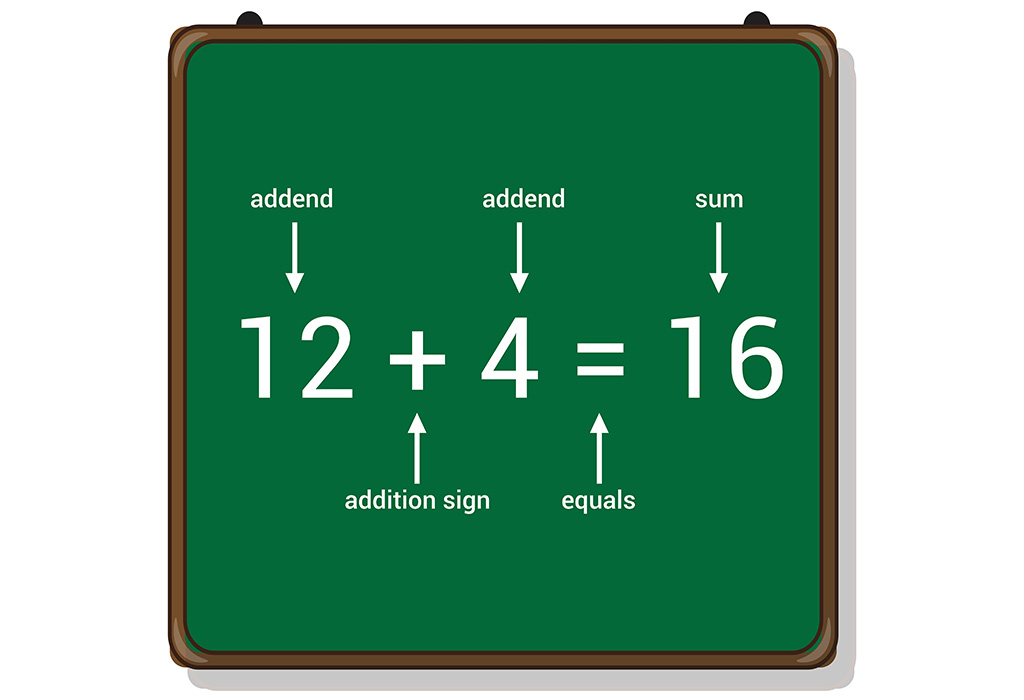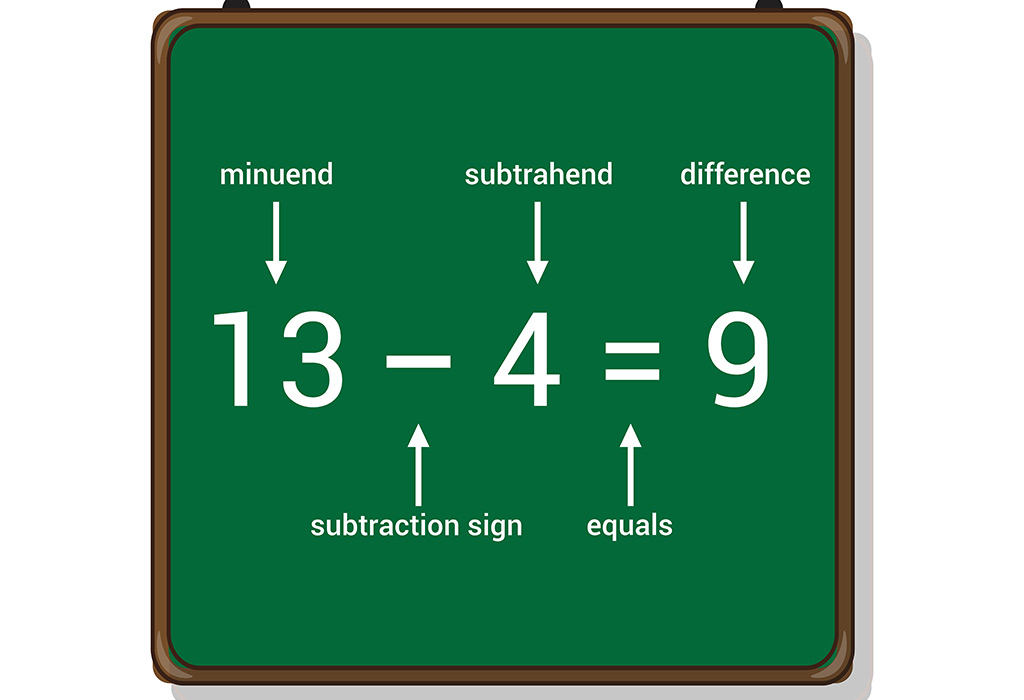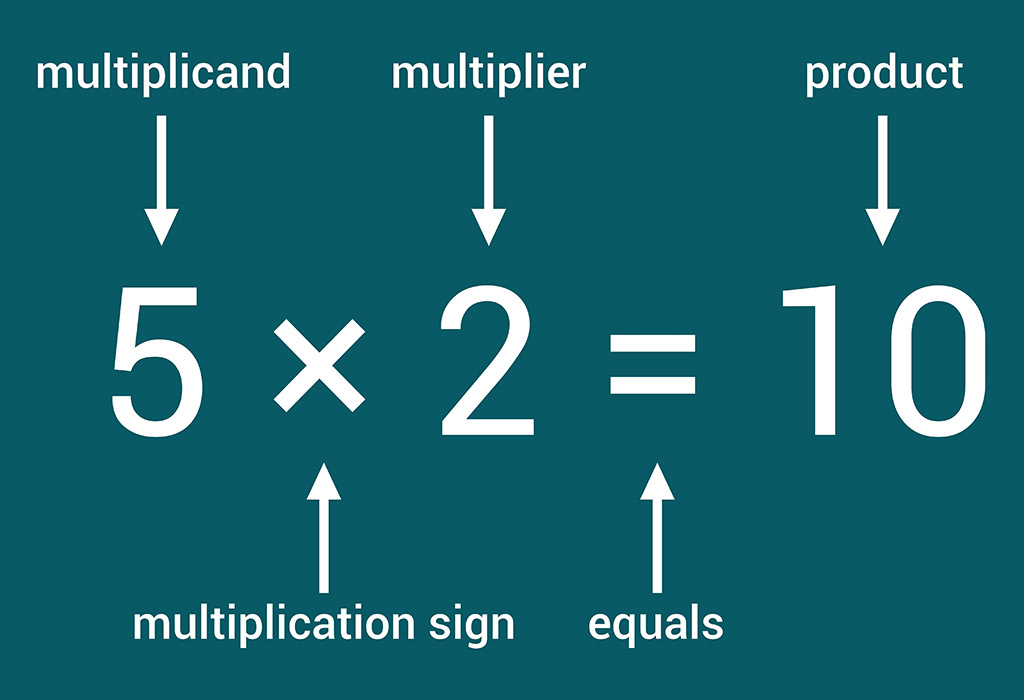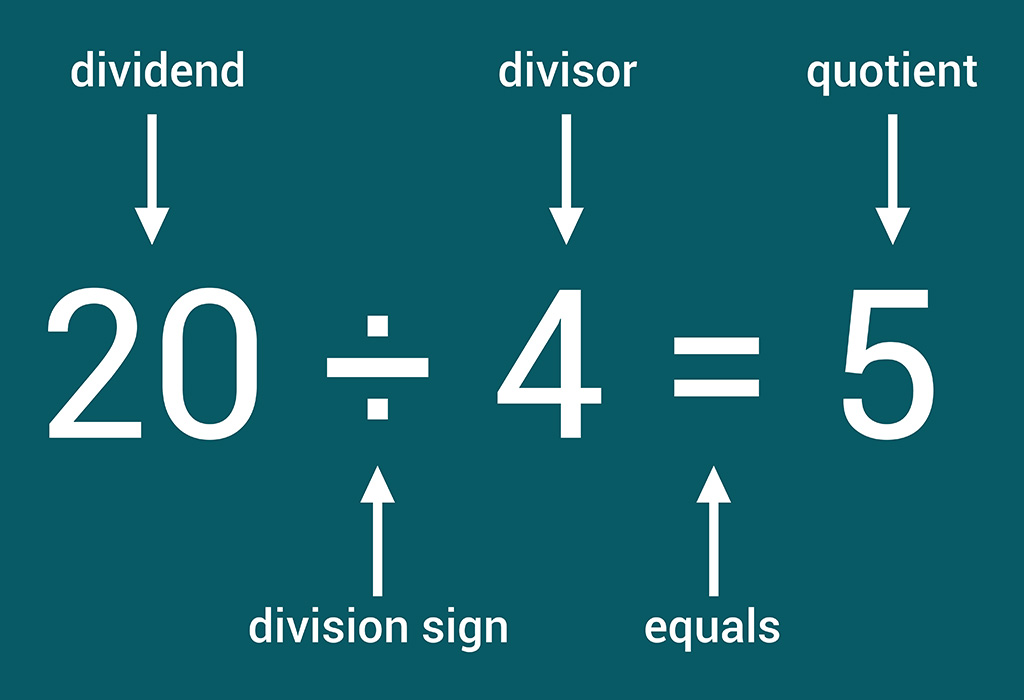HomeBig KidLearning & EducationIntroducing Number Sentence To Preschoolers And Kids With Fun Activities

# Introducing Number Sentence To Preschoolers And Kids With Fun Activities

We all understand how important it is to help children learn to read and write number sentences. When a child understands how a number sentence in maths works, they are not only more familiar with the four basic operations, but they are taking a big step toward understanding the patterns and relationships they will need as they learn to do algebra later in their academic life.

Let’s look at the number of sentence for kindergarten kids.

## What Are Number Sentence?

It is an arrangement of numbers and symbols, referred to as a “sum” or “problem”. Children should be taught about number sentences early as it will help them later in maths lessons. The number sentences can also be defined as the language of mathematics.

Here are some examples of number sentences for children

1. Addition Sentences: 12 + 4 = 162. Subtraction Sentences: 13 – 4 = 93. Multiplication Sentences: 5 x 2 = 104. Division Sentences: 20/4 = 55. Equality Sentences

6. Inequality Sentences

## Importance Of Number Sentences For Kids

Let’s learn about some benefits of learning about number sentences in English with the help of the following points.

• It helps children to understand algebra.
• It provides flexibility to solve simple and complex problems as compared to basic algorithms.

## When Should Your Child Start Learning Number Sentences

• Year 1

Here a child will learn to write and interpret mathematical statements that involves addition, subtraction and equals signs.

• Year 2

Here a child calculates mathematical statements for division and multiplication within the multiplication tables and writes them using the signs.

• Year 3

Here a child learns to write and calculate mathematical statements for multiplication and division using multiplication tables.

• Year 4

Here a child writes statements about the equality of expressions.

• Year 5

Here a child is expected to understand the terms factor, multiple and prime, square and cube numbers and use them to construct statements.

• Year 6

Here a child continues to use all the multiplication tables to calculate mathematical statements to maintain fluency.

## Solved Examples Of Number Sentences For Kids

Below are some solved examples of number sentence examples for kids to practice.

1. Check whether the following sentence is true or false.

12 + 12 + 12 < 4 x 12

Solution: The expression on the left side of the inequality sign is equal to 36.
The expression on the right side of the inequality sign is equal to 48.
48 is greater than 36. Therefore, the statement is true.

2. Complete the sentence so that it is true:

6 + 7 = 9 + ?

Solution: 6 + 7 = 13

And 13 – 9 = 4
Therefore, 6 +7 = 9 + 4

3. Substitute the value into the X and state whether the resulting sentence is true or false.

12 – X = 9
Substitute 5 for X

Solution: Substituting 5 for X in the given sentence, 12 – 5 = 7, which is false, as 12 – 5 = 7, which is not equal to 9.

4. Find the value of the Y so that the following sentence is true.

Y – 24 = 10

Solution: When we add the same number to both sides of the equal sign, it will keep the sentence true. To find the value of Y, we can add 24 to both sides of the equal sign.
Therefore, Y – 24 + 24 = 10 + 24
Y = 34

5. When 6 times a number is increased by 4, the result is 40. Find the number.

Solution: Let X be the number

6 X + 4 = 40
Subtracting 4 from each side gives

6 X = 36
Dividing 36 by 6 gives us

X = 6
So, the number is 6.

6. We have 3 cartons. Each carton contains 3 chocolates. How many total chocolates do we have in 3 cartons?

Solution: 3 times 3 = 9

So, we have 9 chocolates in total.

7. There are 5 butterflies with 02 wings each. How many wings altogether?

Solution: 5 times 2 = 10

So, there are 10 wings in total.

8. I saw 4 tigers today, and the next day I saw 5 tigers. How many tigers have I seen in total?

Solution: 5 + 4 = 9

Therefore, I saw 9 tigers in total.

9. We fed 10 cats today and 20 cats the next day. How many dogs did we feed in 2 days?

Solution: 10 + 20 = 30

Therefore, we fed 30 dogs in total.

10. I presented 7 roses today and 10 roses tomorrow. How many roses did I deliver in 02 days?

Solution: 7 + 10 = 17

Therefore, I delivered 17 roses in 2 days.

## Ways To Teach Number Sentences For Preschoolers

Do you find it difficult to teach your little one multiplication, division, addition or subtraction number sentences? The following tips might be helpful in teaching your children about number sentences.

### 1. Dice Roll

Ask your child to roll a dice and note down the number. Again, ask your child to roll the dice and note down the number with an addition or subtraction sign between the two numbers. Now, ask them to add the numbers.

### 3. Building Blocks

Give your child a bucket of building blocks and ask them to arrange them in stacks according to colour. Now ask them to count and write down the total number of each stack and add them to get the total number of blocks.

RELATED ARTICLES